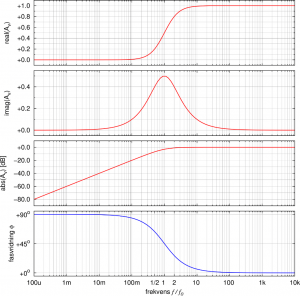# About filters and cut-off frequencies

A good question was brought up by my students in the lab exercise in the course Electronics I yesterday: What actually makes the cut-off frequency of a filter circuit (1st order) so special? Why do we look out for a point which happens to be located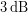or a factor of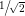under the amplitude of the pass band? Why not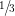,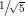,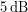?

The first answer, which has some historical relevance, would be to look at a resistor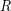and a signal with an amplitude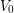and another signal with an amplitude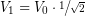while calculating the electrical power: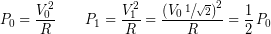The power has been reduced to half the original power, it could be the electric power which is converted to sound waves in a speaker, or the power converted to electromagnetic waves in an antenna.

But already here we can make one important observation: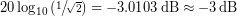Is this everything then? It still sounds random to choose exactly this point. If we for example look at a simple RC filter then we can show another reason which makes this point’s frequency so special. In a low-pass filter we have a constant amplitude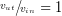for low frequencies while we observed mathematically and experimentally that the amplitude drops with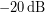per decade of frequency for high frequencies. If we draw out these two asymptotic lines in a Bode diagram, then we will find exactly one point where the lines intersect – you guessed it: it’s at the cut-off frequency (the same holds of course true for a high-pass filter). We can see this if we zoom into the area around the cut-off frequency in a Bode diagram: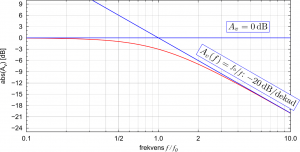Bodediagram of a low-pass filter – zoomed into the area around the cut-off frequency.

As we also saw we have a phase angle of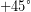(high-pass) or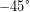(low-pass) between the input- and the output signal at the cut-off frequency. In the complex notation of the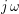-method this correlates to the fact that the real and the imaginary part of the transfer function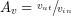have the same magnitude at this point. The relation between real part, imaginary part, amplitude and phase angle I have plotted below for both the low-pass and the high-pass filter.

It is interesting to observe that the imaginary part actually reaches a minimum at this point (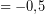for the low-pass filter) or a maximum (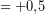for the high-pass filter), respectively.

You can see that the cut-off frequency actually is a remarkable point for a filter circuit.

Low-pass filter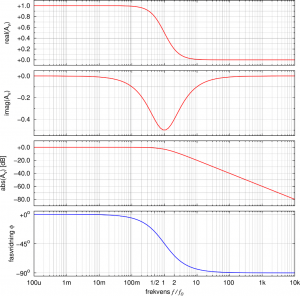High-pass filter